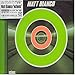## Euler and continued fractions

OK, this one is going to be short, because quite honestly I cannot follow the proof in detail at all. Either I don’t have the required continued fraction mathematics, or I can’t follow advanced calculus proofs any more. Probably option C: all of the above. For a quick refresh of what continued fractions are, see here and here.Leonhard Euler was – quite possibly – the greatest mathematician who ever lived. If not the greatest, he and Gauss could at least duke it out for the top spot. Clifford Truesdell, a historian of science and mathematics, had this to say: “in a listing of all of the mathematics, physics, mechanics, astronomy, and navigation work produced in the 18th century, a full 25% would have been written by Leonhard Euler.” So, he’s, like, way up there.

One of the results Euler proved was that e, the base of natural logarithms, 2.718281828…, was an irrational number. That is, e cannot be expressed as a fraction p/q where p and q are both whole numbers. And he proved it using continued fractions.

Recall that the continued fraction expansion of a rational number is finite. This is essentially proved using Euclid’s Greatest Common Divisor algorithm (which is used to calculate the denominators), where the steps of the algorithm are structured such that the divisors and remainders are always getting smaller and smaller until the remainder reaches 0. Hence, if a continued fraction is infinite, it implies that the equivalent number is not rational. Euler showed that the continued fraction expansion of e was infinite.

One of the examples he gave was that (e – 1)/2 = 0.8591409142295… Taking that approximation as 8,591,409,142,295/10,000,000,000,000, he showed (without a calculator, note!) that

(e – 1)/2 = [0; 1, 6, 10, 14, 18, 22, …]

From this he asserted that the denominators in the continued fraction expansion for e formed an arithmetic progression (each was four more than the previous one, apart from right at the beginning). From this insight and from a more general conclusion about arithmetically progressing denominators derived from a differential equation known as the Ricatti equation, he was able to prove that (e – 1)/2 does in fact equal that infinite continued fraction. Since the continued fraction is infinitely long, it follows that e is irrational.

He found not only that particular expansion for e, but also this one:

e = [2; 1, 2, 1, 1, 4, 1, 1, 6, 1, 1, 8, 1, 1, 10, 1, …]

where I’ve separated out the progression/repeating triads for more visibility. (Donald Knuth expresses this as [1; 0, 1, 1, 2, 1, 1, 4, 1, …] to emphasize the pattern even more. See The Art of Computer Programming, section 4.5.3, especially exercise 16.)

You must admit this simple pattern in the expansion for e is a quite incredible result, especially when you consider that the expansion for ? shows no pattern at all.Now playing:
Matt Bianco - Echoes
(from Echoes)Loading links to posts on similar topics...

#### No Responses

Feel free to add a comment...

##### Leave a response

Note: some MarkDown is allowed, but HTML is not. Expand to show what's available.

•  Emphasize with italics: surround word with underscores `_emphasis_`
•  Emphasize strongly: surround word with double-asterisks `**strong**`
•  Link: surround text with square brackets, url with parentheses `[text](url)`
•  Inline code: surround text with backticks ``IEnumerable``
•  Unordered list: start each line with an asterisk, space `* an item`
•  Ordered list: start each line with a digit, period, space `1. an item`
•  Insert code block: start each line with four spaces
•  Insert blockquote: start each line with right-angle-bracket, space `> Now is the time...`